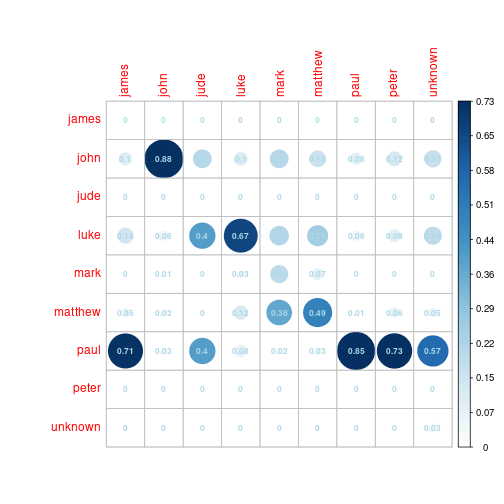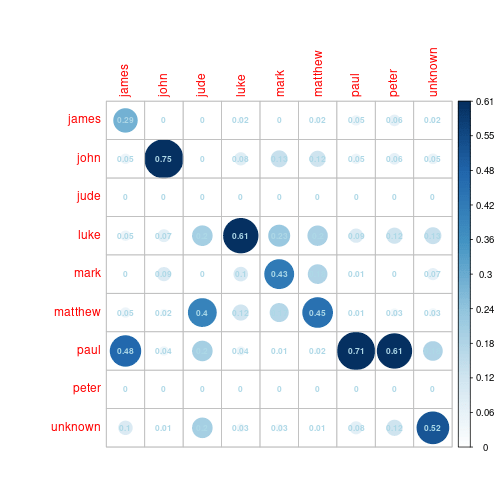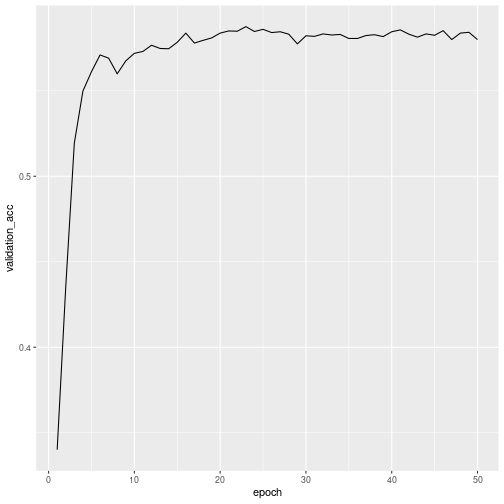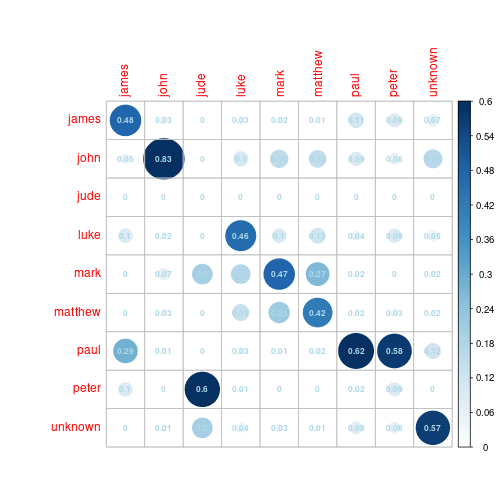Want to share your content on R-bloggers? click here if you have a blog, or here if you don't.

## Introduction

This is the first of what I am hoping are a number of posts on different machine learning classifiers. The subject matter is not lab medicine but the methodology applies to any similar project. For example, maybe you want to classify the text of a general internal medicine consult into its subspecialty based on the words used or perhaps you want to determine which IT tickets are likely high priority. Maybe you want to convert free text diagnoses into categorical diagnoses. Ultimately, the problem I want to tackle is text classification.

In any case, the book that I have been reading at home is Deep Learning with R by Francois Chollet JJ Allaire and given the many interesting and easy-to-follow examples. Since it’s on my mind, I thought a deep learning model would be a good place to start. But I did not want to just redo one of the examples from the book because the data sets are already cleansed and in that sense much of the heavy lifting is done. I wanted to start from a new data set and use the approach shown in section 3.5 but apply it to a new text classification problem. Because I want to follow the basic flow of the Reuters News Wire classifier, I need a similar natural language processing (NLP) multiclass text classifier problem.

The problem I have chosen is one of for authorship classification. Specifically, given any Greek sentence take from the New Testament, can I make a deep learning classifier that will identify the author of a verse that the classifier has never seen?

## Data Cleansing

The text of the New Testament is available online from numerous sources. I downloaded it here and chose the Byzantine Textform 2005 file. The text has been cleansed, put to lower case and transliterated to English characters. There are several steps to get it to a simple dataframe which the following code achieves. The code makes a dataframe where each row is a verse.

```library(tidyverse)
library(janitor)
library(keras)
library(knitr)

# Deals with carriage returns within verses and brings all verses to one row
versify <- function(text, book) {
book_text <- tibble(reference = rep(NA,10000), verse = rep(NA,10000), book = book)
chap_verse_pattern <- "[:space:]*[0-9]{1,3}[:]{1}[0-9]{1,3}[:space:]*"
chap_verse_indices <- str_which(text, chap_verse_pattern)
chap_verse_indices <- c(1, chap_verse_indices)
for(i in 1:(length(chap_verse_indices) - 1)){
verse_text <- paste(text[chap_verse_indices[i] : (chap_verse_indices[i + 1] - 1)], collapse = " ")
reference <- str_extract(verse_text, chap_verse_pattern) %>% str_trim
verse_text <- str_replace(verse_text, chap_verse_pattern, "")
book_text\$verse[i] <- verse_text
if(length(reference) == 0){
book_text\$reference[i] <- NA
} else {
book_text\$reference[i] <- reference
}
}
book_text <- book_text %>%
filter(verse != "") %>%
na.omit()
return(book_text)
}

# read in the books and get the in the expected order just for readability
books <- list.files("Greek/", pattern = ".ASC")
book_order <- c("MT05.ASC",
"MR05.ASC",
"LU05.ASC",
"JOH05.ASC",
"AC05.ASC",
"RO05.ASC",
"1CO05.ASC",
"2CO05.ASC",
"GA05.ASC",
"EPH05.ASC",
"PHP05.ASC",
"COL05.ASC",
"1TH05.ASC",
"2TH05.ASC",
"1TI05.ASC",
"2TI05.ASC",
"TIT05.ASC",
"PHM05.ASC",
"HEB05.ASC",
"JAS05.ASC",
"1PE05.ASC",
"2PE05.ASC",
"1JO05.ASC",
"2JO05.ASC",
"3JO05.ASC",
"JUDE05.ASC",
"RE05.ASC")
# sort book names
book_names <- sort(factor(books, levels = book_order))

# set up author vector
authors <- c("matthew",
"mark",
"luke",
"john",
"luke",
rep("paul", 13),
"unknown",
"james",
rep("peter", 2),
rep("john", 3),
"jude",
"john")

# book author dataframe
authors <- tibble(book = book_names, author = authors)

# prepare empty tibble to store text
nt_frame <- tibble(reference = character(), verse = character(), book = character())

for(i in 1:length(book_names)){
tmp <- versify(tmp, books[i])
nt_frame <- bind_rows(nt_frame,tmp)
}

nt_frame <- left_join(nt_frame, authors, by = "book")

# force correct display order
nt_frame\$book <- factor(nt_frame\$book, levels = book_names)
nt_frame <- arrange(nt_frame, book)```

Now that this wrangling is complete, we have a tibble that looks like this:

```# show tibble format

reference verse book author
1:1 biblov genesewv ihsou cristou uiou dauid uiou abraam MT05.ASC matthew
1:2 abraam egennhsen ton isaak isaak de egennhsen ton iakwb iakwb de egennhsen ton ioudan kai touv adelfouv autou MT05.ASC matthew
1:3 ioudav de egennhsen ton farev kai ton zara ek thv yamar farev de egennhsen ton esrwm esrwm de egennhsen ton aram MT05.ASC matthew
1:4 aram de egennhsen ton aminadab aminadab de egennhsen ton naasswn naasswn de egennhsen ton salmwn MT05.ASC matthew
1:5 salmwn de egennhsen ton booz ek thv racab booz de egennhsen ton wbhd ek thv rouy wbhd de egennhsen ton iessai MT05.ASC matthew
1:6 iessai de egennhsen ton dauid ton basilea dauid de o basileuv egennhsen ton solomwna ek thv tou ouriou MT05.ASC matthew
1:7 solomwn de egennhsen ton roboam roboam de egennhsen ton abia abia de egennhsen ton asa MT05.ASC matthew
1:8 asa de egennhsen ton iwsafat iwsafat de egennhsen ton iwram iwram de egennhsen ton ozian MT05.ASC matthew
1:9 oziav de egennhsen ton iwayam iwayam de egennhsen ton acaz acaz de egennhsen ton ezekian MT05.ASC matthew
1:10 ezekiav de egennhsen ton manassh manasshv de egennhsen ton amwn amwn de egennhsen ton iwsian MT05.ASC matthew

We should get verse counts that match what is expected, which we do.

```# sanity check the verse counts by book
verse_counts <- nt_frame %>%
group_by(book) %>%
summarise(counts = n())
kable(verse_counts)```

book counts
MT05.ASC 1070
MR05.ASC 677
LU05.ASC 1149
JOH05.ASC 878
AC05.ASC 1003
RO05.ASC 432
1CO05.ASC 436
2CO05.ASC 256
GA05.ASC 148
EPH05.ASC 154
PHP05.ASC 103
COL05.ASC 94
1TH05.ASC 88
2TH05.ASC 46
1TI05.ASC 112
2TI05.ASC 82
TIT05.ASC 45
PHM05.ASC 24
HEB05.ASC 302
JAS05.ASC 107
1PE05.ASC 104
2PE05.ASC 60
1JO05.ASC 104
2JO05.ASC 12
3JO05.ASC 13
JUDE05.ASC 24
RE05.ASC 403

And we can check the unique word count

```# check unique words
word_list <- paste(nt_frame\$verse, collapse = " ") %>%
str_split(" ") %>%
unlist %>%
str_replace("[:punct:]","") %>%
tolower()
length(unique(word_list))```

`##  17156`

Normally at this point, we might remove stop words and then stem and lemmatize the text (ie get rid useless little words and suffixes that cause words of the same meaning to look different). This would be more important in more traditional learning classifiers but is likely less important when using Keras and Tensorflow. If I were running this classifier on the English text of the KJV for example, I would run it with and without such a process and guage the performance change. There are numerous NLP packages specifically dedicated to this task. I am going to skip it here. This process is, of course, highly language-dependent.

The other thing I need to do is make the author-factor column numbered 0-8 instead of 1-9 because R is going to be calling python code and python starts counting a 0. This bug took me a while to sort out.

```nt_frame <- nt_frame %>%
mutate(author_factor = as.numeric(as.factor(nt_frame\$author)) - 1) %>% #pythonic
mutate(verse_number  = 1:nrow(nt_frame))```

Now we will make a tokenizer, that is a function to convert words to integers and we will limit the model to the top 15000 out of the 17156 unique words found in the text.

```max_features <- 15000
text <- nt_frame\$verse
tokenizer <- text_tokenizer(num_words = max_features) %>%
fit_text_tokenizer(text)```

Now we need to split the text randomly into training and testing sets in an 80:20 split.

```set.seed(316)
# Select random indices for training using 80% of the data. Notice that these are random!
training_id <- sample.int(nrow(nt_frame), size = nrow(nt_frame)*0.8)
# for reference separate training from testing data
train_data <- nt_frame[training_id,]
test_data <- nt_frame[-training_id,]```

The data is very imbalanced, that is, there are authors (like Jude and James) that have very few verses ascribed to them and there are others (like Paul and Luke) who have many verses. For this reason, we should sanity check our training and testing data to make sure that we have sampled about 80% of each book. There are specific tools to achieve this process which is referred to as stratified sampling.

```train_n <- table(train_data\$author_factor)
test_n <- table(test_data\$author_factor)
train_props <- round(train_n/(train_n + test_n),2)
train_props```

```##
##    0    1    2    3    4    5    6    7    8
## 0.78 0.79 0.67 0.81 0.81 0.81 0.79 0.80 0.81```

We can see that we have a problem with author 2 who has only 24 verses. This is probably not going to matter much but we can try balanced sampling for which we do get better proportions.

```# do balanced sampling
library(splitstackshape)
# get the balanced sample
train_data <- stratified(nt_frame, "author_factor", .8)
# randomize the sample
train_data <- train_data[sample(nrow(train_data)),]
training_id <- train_data\$verse_number

# the test indices are therefore
testing_id <- which(!(nt_frame\$verse_number %in% training_id))

# randomize the sample
test_data <- nt_frame[testing_id,]
test_data <- test_data[sample(nrow(test_data)),]

train_n <- table(train_data\$author_factor)
test_n <- table(test_data\$author_factor)

train_props <- round(train_n/(train_n + test_n),2)
train_props```

```##
##    0    1    2    3    4    5    6    7    8
## 0.80 0.80 0.79 0.80 0.80 0.80 0.80 0.80 0.80```

Now we can tokenize the data, that is, convert the verse from lists of integers to a one-hot encoded form.

```# Create the training and testing x data
x_train <- texts_to_matrix(tokenizer, text[training_id], mode = "binary")
x_test <- texts_to_matrix(tokenizer, text[-training_id], mode = "binary")

# Set the training and testing y data and then one hot encode them
train_labels <- nt_frame\$author_factor[training_id]
y_train <- to_categorical(train_labels)

test_labels <- nt_frame\$author_factor[-training_id]
y_test <- to_categorical(test_labels)```

Satisfy ourselves that the training data is random in order

`kable(head(nt_frame[training_id,], 10))`

reference verse book author author_factor verse_number
16:6 all oti tauta lelalhka umin h luph peplhrwken umwn thn kardian JOH05.ASC john 1 3584
2:20 all ecw kata sou oti afeiv thn gunaika sou iezabel h legei eauthn profhtin kai didaskei kai plana touv emouv doulouv porneusai kai fagein eidwloyuta RE05.ASC john 1 7563
21:23 kai elyonti autw eiv to ieron proshlyon autw didaskonti oi arciereiv kai oi presbuteroi tou laou legontev en poia exousia tauta poieiv kai tiv soi edwken thn exousian tauthn MT05.ASC matthew 5 705
5:1 dikaiwyentev oun ek pistewv eirhnhn ecomen prov ton yeon dia tou kuriou hmwn ihsou cristou RO05.ASC paul 6 4895
12:29 h pwv dunatai tiv eiselyein eiv thn oikian tou iscurou kai ta skeuh autou diarpasai ean mh prwton dhsh ton iscuron kai tote thn oikian autou diarpasei MT05.ASC matthew 5 374
4:24 alla kai di hmav oiv mellei logizesyai toiv pisteuousin epi ton egeiranta ihsoun ton kurion hmwn ek nekrwn RO05.ASC paul 6 4893
27:31 eipen o paulov tw ekatontarch kai toiv stratiwtaiv ean mh outoi meinwsin en tw ploiw umeiv swyhnai ou dunasye AC05.ASC luke 3 4734
1:25 kai hrwthsan auton kai eipon autw ti oun baptizeiv ei su ouk ei o cristov oute hliav oute o profhthv JOH05.ASC john 1 2921
3:6 kai exelyontev oi farisaioi euyewv meta twn hrwdianwn sumboulion epoioun kat autou opwv auton apoleswsin MR05.ASC mark 4 1149
8:4 ei men gar hn epi ghv oud an hn iereuv ontwn twn ierewn twn prosferontwn kata ton nomon ta dwra HEB05.ASC unknown 8 6930

Now we can build a basic model:

```# Build a basic model
model <- keras_model_sequential() %>%
layer_dense(units = 64, activation = "relu", input_shape = c(max_features)) %>%
layer_dense(units = 64, activation = "relu") %>%
layer_dense(units = 9, activation = "softmax")

model %>% compile(
optimizer = "rmsprop",
loss = "categorical_crossentropy",
metrics = c("accuracy")
)```

and pull out validation data, again in an 80:20 split.

```# pull out some validation data
val_indices <- 1:floor(nrow(train_data))*0.2
x_val <- x_train[val_indices,]
partial_x_train <- x_train[-val_indices,]
y_val <- y_train[val_indices,]
partial_y_train = y_train[-val_indices,]```

Now we run the model:

```history <- model %>% keras::fit(
partial_x_train, # train in the non-validation training data
partial_y_train,
epochs = 5,
batch_size = 256,
validation_data = list(x_val, y_val)
)

results <- model %>%
keras::evaluate(x_test, y_test)
results```

```##      loss  accuracy
## 1.0402801 0.6382576```

```plot(history) +
geom_line()```We can show the model performance graphically:

```library(corrplot)
pred <- model %>%
predict_classes(x_test) %>%
factor(0:8)

res_tab <- table(Pred = pred, Act = test_labels)
res_prop <- prop.table(res_tab,2)

author_key <- tibble(author = nt_frame\$author, code = nt_frame\$author_factor) %>%
unique %>%
arrange(code)

colnames(res_prop) <- author_key\$author
rownames(res_prop) <- author_key\$author
corrplot(res_prop,is.corr = FALSE,
method = "circle", addCoef.col = "lightblue", number.cex = 0.7)```Results are not great because many authors are being misclassified as Paul or Luke. This is likely from author imbalance so we can address the imbalance with weights and with dropout layers as suggested in this very informative tutorial from
Dr. Bharatendra Rai
.

```model <- keras_model_sequential() %>%
layer_dense(units = 64, activation = "relu", input_shape = c(max_features)) %>%
layer_dropout(rate = 0.4) %>%
layer_dense(units = 32, activation = 'relu') %>%
layer_dropout(rate = 0.2) %>%
layer_dense(units = 9, activation = "softmax")

model %>%
compile(loss = 'categorical_crossentropy',
optimizer = 'rmsprop',
metrics = 'accuracy')

history <- model %>% keras::fit(
partial_x_train,
partial_y_train,
epochs = 5,
batch_size = 256,
validation_data = list(x_val, y_val),
class_weight = list("0" = 20.1, "1" = 1.5, "2" = 89.7, "3" = 1.0, "4" = 3.2, "5" = 2.0, "6" = 1.1, "7" = 13.1, "8" = 7.1))

results <- model %>% keras::evaluate(x_test, y_test)
results```

```##      loss  accuracy
## 1.4781048 0.5997475```

```pred <- model %>%
predict_classes(x_test) %>%
factor(0:8)
# res_frame <- tibble(Prediction = pred, Target = test_labels)
# res_frame <- res_frame %>%
#   group_by(Prediction, Target) %>%
#   summarise(N = n())
res_tab <- table(Pred = pred, Act = test_labels)
res_tab```

```##     Act
## Pred   0   1   2   3   4   5   6   7   8
##    0   6   1   0   8   0   4  19   2   1
##    1   1 211   0  34  18  26  19   2   3
##    2   0   1   0   2   0   0   1   0   0
##    3   1  21   1 261  31  42  38   4   8
##    4   0  26   0  42  58  39   5   0   4
##    5   1   7   2  52  23  97   5   1   2
##    6  10  11   1  16   1   4 286  20  11
##    7   0   0   0   1   0   0   0   0   0
##    8   2   4   1  14   4   2  31   4  31```

```res_prop <- prop.table(res_tab,2)
colnames(res_prop) <- author_key\$author
rownames(res_prop) <- author_key\$author```

What we get looks a little better with more counts on the diagonal.

```corrplot(res_prop,is.corr = FALSE,
method = "circle", addCoef.col = "lightblue", number.cex = 0.7)```The model is jumpy on the small books, probably because of undersampling of them. This means that k-fold cross validation help us assess model performance. Not sure if I should try to have balanced sampling in the folds but I am not going to worry about that at the moment.

```build_model <- function() {
model <- keras_model_sequential() %>%
layer_dense(units = 64, activation = "relu", input_shape = c(max_features)) %>%
layer_dropout(rate = 0.4) %>%
layer_dense(units = 32, activation = 'relu') %>%
layer_dropout(rate = 0.2) %>%
layer_dense(units = 9, activation = "softmax")

model %>%
compile(loss = 'categorical_crossentropy',
optimizer = 'rmsprop',
metrics = 'accuracy')
}```

Run the k-fold cross-validation.

```acc_histories <- NULL
loss_histories <- NULL
k <- 4
indices <- sample(1:nrow(train_data))
folds <- cut(1:length(indices), breaks = k, labels = FALSE)
num_epochs <- 50
all_scores <- c()
proportions_list <- list()

for (i in 1:k) {
cat("processing fold #", i, "\n")
# Prepare the validation data: data from partition # k
val_indices <- which(folds == i, arr.ind = TRUE)
x_val_kfold <- x_train[val_indices,]
y_val_kfold <- y_train[val_indices,]

# Prepare the training data: data from all other partitions
#partial_train_data <- train_data[-val_indices,]
x_train_kfold <- x_train[-val_indices,]
y_train_kfold <- y_train[-val_indices,]

# Build the Keras model (already compiled)
model <- build_model()

# Train the model (in silent mode, verbose=0)
history <- model %>% fit(x_train_kfold, y_train_kfold,
epochs = num_epochs,
batch_size = 256,
validation_data = list(x_val_kfold, y_val_kfold),
verbose = 0,
class_weight = list("0" = 20.1, "1" = 1.5, "2" = 89.7, "3" = 1.0, "4" = 3.2, "5" = 2.0, "6" = 1.1, "7" = 13.1, "8" = 7.1))

# Evaluate the model on the fold's validation data
results <- model %>% keras::evaluate(x_val_kfold, y_val_kfold)
all_scores <- c(all_scores, results["accuracy"])

pred <- model %>%
predict_classes(x_val_kfold) %>%
factor(0:8)

res_tab <- table(Pred = pred, Act = train_data\$author_factor[val_indices])
res_prop <- prop.table(res_tab,2)
proportions_list[[i]] <- res_prop

acc_history <- history\$metrics\$val_accuracy
acc_histories <- rbind(acc_histories, acc_history)
loss_history <- history\$metrics\$loss
loss_histories <- rbind(loss_history, acc_history)
}

all_scores
mean(all_scores)
average_acc_history <- data.frame(
epoch = seq(1:ncol(acc_histories)),
validation_acc = apply(acc_histories, 2, mean)
)
average_loss_history <- data.frame(
epoch = seq(1:ncol(loss_histories)),
validation_loss = apply(loss_histories, 2, mean)
)```

Validation accuracy improves modestly with more epochs but the model definitely overfits the training data (getting to the high 90s in accuracy). This is a bit of a conundrum to me for which I do not know the answer (those who know, please comment): namely, I can overfit the model to make gains on the validation set and these do improve performance on the test set but I expect that this improvement is happening in some non-generalizable way.

```library(ggplot2)
ggplot(average_acc_history, aes(x = epoch, y = validation_acc)) + geom_line()```Likewise loss slowly declines over many epochs but the model overfits.

`ggplot(average_loss_history, aes(x = epoch, y = validation_loss)) + geom_line()`In any case, this is the model performance rerunning the k-fold cross validation with 5 epochs.

## Final Outcome

Satisfied enough that 5 epochs should be OK, I can run the model on the whole training set and look at its performance on the testing set.

```model <- keras_model_sequential() %>%
layer_dense(units = 64, activation = "relu", input_shape = c(max_features)) %>%
layer_dropout(rate = 0.4) %>%
layer_dense(units = 32, activation = 'relu') %>%
layer_dropout(rate = 0.2) %>%
layer_dense(units = 9, activation = "softmax")

model %>%
compile(loss = 'categorical_crossentropy',
optimizer = 'rmsprop',
metrics = 'accuracy')

history <- model %>% keras::fit(
x_train,
y_train,
epochs = 5,
batch_size = 256,
#validation_data = list(x_val, y_val),
class_weight = list("0" = 20.1, "1" = 1.5, "2" = 89.7, "3" = 1.0, "4" = 3.2, "5" = 2.0, "6" = 1.1, "7" = 13.1, "8" = 7.1))

results <- model %>% keras::evaluate(x_test, y_test)
results```

```##      loss  accuracy
## 1.4544461 0.5568182```

```pred <- model %>%
predict_classes(x_test) %>%
factor(0:8)
# res_frame <- tibble(Prediction = pred, Target = test_labels)
# res_frame <- res_frame %>%
#   group_by(Prediction, Target) %>%
#   summarise(N = n())
#
res_tab <- table(Pred = pred, Act = test_labels)
res_tab```

```##     Act
## Pred   0   1   2   3   4   5   6   7   8
##    0  10   8   0  15   3   3  45   3   4
##    1   1 233   0  45  21  31  38   2  10
##    2   0   0   0   0   0   0   1   0   0
##    3   2   6   0 198  13  25  17   3   3
##    4   0  19   1  77  64  57   7   0   1
##    5   0   8   0  57  29  90   7   1   1
##    6   6   4   0  14   1   5 250  19   7
##    7   2   0   3   5   0   0   8   3   0
##    8   0   4   1  19   4   3  31   2  34```

```res_prop <- prop.table(res_tab,2)
colnames(res_prop) <- author_key\$author
rownames(res_prop) <- author_key\$author
corrplot(res_prop,is.corr = FALSE,
method = "circle", addCoef.col = "lightblue", number.cex = 0.7)```Some interesting findings:

• John seems to be the easiest to classify. This fits well with his unique authorship style.
• The synoptic gospels are are easily misclassified among one another. Again, this fits with the overlap of stories, parables and other content.
• Hebrews looks more like Hebrews than it looks like Paul. This fits with the perspective that Paul is not the author of Hebrews.
• Poor James, Jude and Peter: just not enough verses to get proper classification. I am sure there are ways to address this kind of imballance were classifying Jude correctly a very important thing to do.

I think I am going to stop trying to improve this because it is not a real problem but I hope that someone else can recycle some code for a real-life problem. I would be interested in comments on how to get improved classification of small classes.

Parting Easter Thought

ouk estin wde hgeryh gar kaywv eipen deute idete ton topon opou ekeito o kuriov, Matthew 28:6